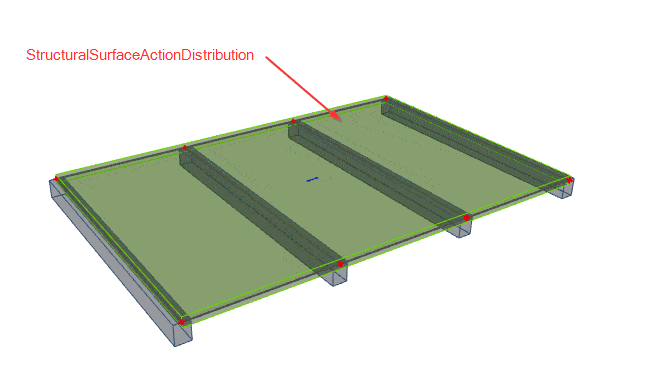# StructuralSurfaceActionDistribution¶

StructuralSurfaceActionDistribution object describes how surface load can be distributed to other members.

Warning

The name of the excel sheet is StructuralSurfaceActionDistri due to sheet name length limitation.## Specification in the excel¶

Data type

Example / enum definition

Required

Description

Name

String

FL1

yes

Human readable unique name of the Arbitrary definition

Type

Enum

Nodes

Edges

Beams and edges

yes

Defines where the load should be distributed.

Nodes - load is expected to be distributed to nodes of StructuralSurfaceActionDistribution

Edges - load is expected to be distributed to edges of StructuralSurfaceActionDistribution

Beams and edges - load is expected to be distributed to edges and specified beams of StructuralSurfaceActionDistribution

Nodes

String

N81; N263; N659; N660

yes

All nodes that belongs to StructuralSurfaceActionDistribution member and defines its geometric shape. The names of the nodes are separated by ; (semicolon) and space.

Edges

String

Line; Line; Circular Arc; Line

or

Line;Spline-5;Line;Parabolic Arc;Line

yes

Defines the shape of the curve between two next nodes (or more nodes depends on edge type). Supported strings are:

• Line
• Circular Arc
• Circle by 3 points
• Circle and Point
• Parabolic arc
• Bezier
• Spline-#

Where “#” number of nodes defining the spline

The names are separated by ; (semicolon) and space. It possible to also define circle geometry by using “Circle and Point” or “Circle by 3 points”. In case of “Circle and point” two nodes have to be defined, centre of the circle and a point on a circle. The circle defined by two points is always horizontal.

Layer

String

no

Custom created layer. The layer can thus comprise entities that have something in common (e.g. one floor, columns of one floor, columns of the same length, etc.)

LCS Type

Enum

x by vector

y by vector

Tilt of vector defined by point

yes

Defines type of the local coordinate system of the StructuralSurfaceActionDistribution. Option “Tilt of vector defined by point” allows change of orientation of the LCS to one point. For this, you have to specify coordinates of the vector.

For further understanding see Introduction

Coordinate X [m]

Double

1

yes

Coordinate of the vector of the LCS in X direction

Coordinate Y [m]

Double

0

yes

Coordinate of the vector of the LCS in Y direction

Coordinate Z [m]

Double

1.2

yes

Coordinate of the vector of the LCS in Z direction

LCS Rotation [deg]

Double

45.00

yes

This value defines the rotation of local axes of the StructuralSurfaceActionDistribution member.

It is angle around Z axis (axis which is perpendicular to plane) and defines distribution angle.

Positive value = anti clock wise.

Distribution to

Enum

One way - X

One way - Y

Two way

yes

Defines how the load will be distributed.

One way - X - Load will be distributed only to members parallel with X direction (X axis of LCS)

One way - Y - Load will be distributed only to members parallel with Y direction (Y axis of LCS)

Two way - Load will be distributed equally to both directions

String

B1 ; B2 ; B3 ; B5

no

Allows to define the subset of loaded beams.

Taken into account only if Type = Beams and edges

If blank = all beams are loaded

Id

String

39f238a5-01d0-45cf-a2eb-958170fd4f39

no

Unique attribute designation